October 24, 2021

Remember Me

### Create an account

Fields marked with an asterisk (*) are required.
Name *
Email *
Verify email *## Overview of major hyperparameter optimization techniques

Machine Learning

User Rating: 0 / 5Whenever we perform any machine learning task, we follow the normal pipeline of preprocessing data, feature engineering choosing the model followed by training and testing

Tags:

## Bayesian optimization in hyperparameter optimization (concepts and implementation)

Machine Learning

User Rating: 0 / 5The term bayesian comes from thomas bayes, a well known statistician from the 18th century, he is famous for establishing the bayes rule.

Tags:

## A Conceptual Explanation of Bayesian Hyperparameter Optimization for Machine Learning

Machine Learning

User Rating: 0 / 5Following are four common methods of hyperparameter optimization for machine learning in order of increasing efficiency:

1. Manual
2. Grid search
3. Random search
4. Bayesian model-based optimization

(There are also other methods such as evolutionary and gradient-based.)

I was pretty proud that I’d recently moved up the ladder from manual to random search until I found this image deep in a paper by Bergstra et al.:These figures compare validation error for hyperparameter optimization of an image classification neural network with random search in grey and Bayesian Optimization (using the Tree Parzen Estimator or TPE) in green. Lower is better: a smaller validation set error generally means better test set performance, and a smaller number of trials means less time invested. Clearly, there are significant advantages to Bayesian methods, and these graphs, along with other impressive results, convinced me it was time to take the next step and learn model-based hyperparameter optimization.

The one-sentence summary of Bayesian hyperparameter optimization is: build a probability model of the objective function and use it to select the most promising hyperparameters to evaluate in the true objective function.

If you like to operate at a very high level, then this sentence may be all you need. However, if you want to understand the details, this article is my attempt to outline the concepts behind Bayesian optimization, in particular Sequential Model-Based Optimization (SMBO) with the Tree Parzen Estimator (TPE). With the mindset that you don’t know a concept until you can explain it to others, I went through several academic papers and will try to communicate the results in a (relatively) easy to understand format.

Although we can often implement machine learning methods without understanding how they work, I like to try and get an idea of what is going on so I can use the technique as effectively as possible. In later articles I’ll walk through using these methods in Python using libraries such as Hyperopt, so this article will lay the conceptual groundwork for implementations to come!

Update: Here is a brief Jupyter Notebook showing the basics of using Bayesian Model-Based Optimization in the Hyperopt Python library.

## Hyperparameter Optimization

The aim of hyperparameter optimization in machine learning is to find the hyperparameters of a given machine learning algorithm that return the best performance as measured on a validation set. (Hyperparameters, in contrast to model parameters, are set by the machine learning engineer before training. The number of trees in a random forest is a hyperparameter while the weights in a neural network are model parameters learned during training. I like to think of hyperparameters as the model settings to be tuned.)

Hyperparameter optimization is represented in equation form as:Here f(x) represents an objective score to minimize— such as RMSE or error rate— evaluated on the validation set; x* is the set of hyperparameters that yields the lowest value of the score, and x can take on any value in the domain X. In simple terms, we want to find the model hyperparameters that yield the best score on the validation set metric.

The problem with hyperparameter optimization is that evaluating the objective function to find the score is extremely expensive. Each time we try different hyperparameters, we have to train a model on the training data, make predictions on the validation data, and then calculate the validation metric. With a large number of hyperparameters and complex models such as ensembles or deep neural networks that can take days to train, this process quickly becomes intractable to do by hand!

Grid search and random search are slightly better than manual tuning because we set up a grid of model hyperparameters and run the train-predict -evaluate cycle automatically in a loop while we do more productive things (like feature engineering). However, even these methods are relatively inefficient because they do not choose the next hyperparameters to evaluate based on previous results. Grid and random search are completely uninformed by past evaluations, and as a result, often spend a significant amount of time evaluating “bad” hyperparameters.

For example, if we have the following graph with a lower score being better, where does it make sense to concentrate our search? If you said below 200 estimators, then you already have the idea of Bayesian optimization! We want to focus on the most promising hyperparameters, and if we have a record of evaluations, then it makes sense to use this information for our next choice.Random and grid search pay no attention to past results at all and would keep searching across the entire range of the number of estimators even though it’s clear the optimal answer (probably) lies in a small region!

## Bayesian Optimization

Bayesian approaches, in contrast to random or grid search, keep track of past evaluation results which they use to form a probabilistic model mapping hyperparameters to a probability of a score on the objective function:In the literature, this model is called a “surrogate” for the objective function and is represented as p(y | x). The surrogate is much easier to optimize than the objective function and Bayesian methods work by finding the next set of hyperparameters to evaluate on the actual objective function by selecting hyperparameters that perform best on the surrogate function. In other words:

1. Build a surrogate probability model of the objective function
2. Find the hyperparameters that perform best on the surrogate
3. Apply these hyperparameters to the true objective function
4. Update the surrogate model incorporating the new results
5. Repeat steps 2–4 until max iterations or time is reached

The aim of Bayesian reasoning is to become “less wrong” with more data which these approaches do by continually updating the surrogate probability model after each evaluation of the objective function.

At a high-level, Bayesian optimization methods are efficient because they choose the next hyperparameters in an informed manner. The basic idea is: spend a little more time selecting the next hyperparameters in order to make fewer calls to the objective function. In practice, the time spent selecting the next hyperparameters is inconsequential compared to the time spent in the objective function. By evaluating hyperparameters that appear more promising from past results, Bayesian methods can find better model settings than random search in fewer iterations.

If there’s one thing to take away from this article it’s that Bayesian model-based methods can find better hyperparameters in less time because they reason about the best set of hyperparameters to evaluate based on past trials.

As a good visual description of what is occurring in Bayesian Optimization take a look at the images below (source). The first shows an initial estimate of the surrogate model — in black with associated uncertainty in gray — after two evaluations. Clearly, the surrogate model is a poor approximation of the actual objective function in red:The next image shows the surrogate function after 8 evaluations. Now the surrogate almost exactly matches the true function. Therefore, if the algorithm selects the hyperparameters that maximize the surrogate, they will likely yield very good results on the true evaluation function.Bayesian methods have always made sense to me because they operate in much the same way we do: we form an initial view of the world (called a prior) and then we update our model based on new experiences (the updated model is called a posterior). Bayesian hyperparameter optimization takes that framework and applies it to finding the best value of model settings!

#### Sequential Model-Based Optimization

Sequential model-based optimization (SMBO) methods (SMBO) are a formalization of Bayesian optimization. The sequential refers to running trials one after another, each time trying better hyperparameters by applying Bayesian reasoning and updating a probability model (surrogate).

There are five aspects of model-based hyperparameter optimization:

1. A domain of hyperparameters over which to search
2. An objective function which takes in hyperparameters and outputs a score that we want to minimize (or maximize)
3. The surrogate model of the objective function
4. A criteria, called a selection function, for evaluating which hyperparameters to choose next from the surrogate model
5. A history consisting of (score, hyperparameter) pairs used by the algorithm to update the surrogate model

There are several variants of SMBO methods that differ in steps 3–4, namely, how they build a surrogate of the objective function and the criteria used to select the next hyperparameters. Several common choices for the surrogate model are Gaussian Processes, Random Forest Regressions, and Tree Parzen Estimators (TPE) while the most common choice for step 4 is Expected Improvement. In this post, we will focus on TPE and Expected Improvement.

#### Domain

In the case of random search and grid search, the domain of hyperparameters we search is a grid. An example for a random forest is shown below:

``````hyperparameter_grid = {
'n_estimators': [100, 200, 300, 400, 500, 600],
'max_depth': [2, 5, 10, 15, 20, 25, 30, 35, 40],
'min_samples_leaf': [1, 2, 3, 4, 5, 6, 7, 8]
}``````

For a model-based approach, the domain consists of probability distributions. As with a grid, this lets us encode domain knowledge into the search process by placing greater probability in regions where we think the true best hyperparameters lie. If we wanted to express the above grid as a probability distribution, it may look something like this:Here we have a uniform, log-normal, and normal distribution. These are informed by prior practice/knowledge (for example the learning rate domain is usually a log-normal distribution over several orders of magnitude).

#### Objective Function

The objective function takes in hyperparameters and outputs a single real-valued score that we want to minimize (or maximize). As an example, let’s consider the case of building a random forest for a regression problem. The hyperparameters we want to optimize are shown in the hyperparameter grid above and the score to minimize is the Root Mean Squared Error. Our objective function would then look like (in Python):

``````import numpy as np
from sklearn.ensemble improt RandomForestRegressor

# Function mapping hyperparameters to a real-valued scpre
def objective(hyperparameters):

# Machine learning model
rf = RandomForestRegressor(**hyperparameters)

# Training
rf.fit(X_train, y_train)

# Making predictions and evaluating
predictions = rf.predict(X_valid)
rmse = np.sqrt(np.mean(np.square(prediction - y_valid)))

return rmse``````

While the objective function looks simple, it is very expensive to compute! If the objective function could be quickly calculated, then we could try every single possible hyperparameter combination (like in grid search). If we are using a simple model, a small hyperparameter grid, and a small dataset, then this might be the best way to go. However, in cases where the objective function may take hours or even days to evaluate, we want to limit calls to it.

The entire concept of Bayesian model-based optimization is to reduce the number of times the objective function needs to be run by choosing only the most promising set of hyperparameters to evaluate based on previous calls to the evaluation function. The next set of hyperparameters are selected based on a model of the objective function called a surrogate.

#### Surrogate Function (Probability Model)

The surrogate function, also called the response surface, is the probability representation of the objective function built using previous evaluations. This is called sometimes called a response surface because it is a high-dimensional mapping of hyperparameters to the probability of a score on the objective function. Below is a simple example with only two hyperparameters:There are several different forms of the surrogate function including Gaussian Processes and Random Forest regression. However, in this post we will focus on the Tree-structured Parzen Estimator as put forward by Bergstra et al in the paper “Algorithms for Hyper-Parameter Optimization”. These methods differ in how they construct the surrogate function which we’ll explain in just a bit. First we need to talk about the selection function.

#### Selection Function

The selection function is the criteria by which the next set of hyperparameters are chosen from the surrogate function. The most common choice of criteria is Expected Improvement:Here y* is a threshold value of the objective function, x is the proposed set of hyperparameters, y is the actual value of the objective function using hyperparameters x, and p(y | x) is the surrogate probability model expressing the probability of y given x. If that’s all a little much, in simpler terms, the aim is to maximize the Expected Improvement with respect to x. This means finding the best hyperparameters under the surrogate function p (y | x).

If p (y | x) is zero everywhere that y < y*, then the hyperparameters x are not expected to yield any improvement. If the integral is positive, then it means that the hyperparameters x are expected to yield a better result than the threshold value.

Tree-structured Parzen Estimator (TPE)

Now let’s get back to the surrogate function. The methods of SMBO differ in how they construct the surrogate model p(y | x). The Tree-structured Parzen Estimator builds a model by applying Bayes rule. Instead of directly representing p( y | x), it instead uses:p (x | y), which is the probability of the hyperparameters given the score on the objective function, in turn is expressed:where y < y* represents a lower value of the objective function than the threshold. The explanation of this equation is that we make two different distributions for the hyperparameters: one where the value of the objective function is less than the threshold, l(x), and one where the value of the objective function is greater than the threshold, g(x).

Let’s update our Random Forest graph to include a threshold:Now we construct two probability distributions for the number of estimators, one using the estimators that yielded values under the threshold and one using the estimators that yielded values above the threshold.Intuitively, it seems that we want to draw values of x from l(x) and not from g(x) because this distribution is based only on values of x that yielded lower scores than the threshold. It turns out this is exactly what the math says as well! With Bayes Rule, and a few substitutions, the expected improvement equation (which we are trying to maximize) becomes:The term on the far right is the most important part. What this says is that the Expected Improvement is proportional to the ratio l(x) / g(x) and therefore, to maximize the Expected Improvement, we should maximize this ratio. Our intuition was correct: we should draw values of the hyperparameters which are more likely under l(x) than under g(x)!

The Tree-structured Parzen Estimator works by drawing sample hyperparameters from l(x), evaluating them in terms of l(x) / g(x), and returning the set that yields the highest value under l(x) / g(x) corresponding to the greatest expected improvement. These hyperparameters are then evaluated on the objective function. If the surrogate function is correct, then these hyperparameters should yield a better value when evaluated!

The expected improvement criteria allows the model to balance exploration versus exploitation. l(x) is a distribution and not a single value which means that the hyperparameters drawn are likely close but not exactly at the maximum of the expected improvement. Moreover, because the surrogate is just an estimate of the objective function, the selected hyperparameters may not actually yield an improvement when evaluated and the surrogate model will have to be updated. This updating is done based on the current surrogate model and the history of objective function evaluations.

#### History

Each time the algorithm proposes a new set of candidate hyperparameters, it evaluates them with the actual objective function and records the result in a pair (score, hyperparameters). These records form the history. The algorithm builds l(x) and g(x) using the history to come up with a probability model of the objective function that improves with each iteration.

This is Bayes’ Rule at work: we have an initial estimate for the surrogate of the objective function that we update as we gather more evidence. Eventually, with enough evaluations of the objective function, we hope that our model accurately reflects the objective function and the hyperparameters that yield the greatest Expected Improvement correspond to the hyperparameters that maximize the objective function.

## Putting it All Together

How do Sequential Model-Based Methods help us more efficiently search the hyperparameter space? Because the algorithm is proposing better candidate hyperparameters for evaluation, the score on the objective function improves much more rapidly than with random or grid search leading to fewer overall evaluations of the objective function.

Even though the algorithm spends more time selecting the next hyperparameters by maximizing the Expected Improvement, this is much cheaper in terms of computational cost than evaluating the objective function. In a paper about using SMBO with TPE, the authors reported that finding the next proposed set of candidate hyperparameters took several seconds, while evaluating the actual objective function took hours.

If we are using better-informed methods to choose the next hyperparameters, that means we can spend less time evaluating poor hyperparameter choices. Furthermore, sequential model-based optimization using tree-structured Parzen estimators is able to find better hyperparameters than random search in the same number of trials. In other words, we get

• Reduced running time of hyperparameter tuning
• Better scores on the testing set

Hopefully, this has convinced you Bayesian model-based optimization is a technique worth trying!

#### Implementation

Fortunately for us, there are now a number of libraries that can do SMBO in Python. Spearmint and MOE use a Gaussian Process for the surrogate, Hyperopt uses the Tree-structured Parzen Estimator, and SMAC uses a Random Forest regression. These libraries all use the Expected Improvement criterion to select the next hyperparameters from the surrogate model. In later articles we will take a look at using Hyperopt in Python and there are already several good articles and code examples for learning.

## Conclusions

Bayesian model-based optimization methods build a probability model of the objective function to propose smarter choices for the next set of hyperparameters to evaluate. SMBO is a formalization of Bayesian optimization which is more efficient at finding the best hyperparameters for a machine learning model than random or grid search.

Sequential model-based optimization methods differ in they build the surrogate, but they all rely on information from previous trials to propose better hyperparameters for the next evaluation. The Tree Parzen Estimator is one algorithm that uses Bayesian reasoning to construct the surrogate model and can select the next hyperparameters using Expected Improvement.

There are a number of libraries to implement SMBO in Python which we will explore in further articles. The concepts are a little tough at first, but understanding them will allow us to use the tools built on them more effectively. I’d like to mention I’m still trying to work my way through the details and if I’ve made a mistake, please let me know (civilly)!

For more details, the following articles are extremely helpful:

1. Algorithms for Hyper-Parameter Optimization [Link]
2. Making a Science of Model Search: Hyperparameter Optimization in Hundreds of Dimensions for Vision Architectures [Link]
4. Taking the Human Out of the Loop: A Review of Bayesian Optimization [Link]

Author:

Will Koehrsen
Data Scientist at Cortex Intel, Data Science Communicator

## Get started with: Decision Tree, Random Forest, and XGBoost.

Machine Learning

User Rating: 0 / 5# Introduction

This tutorial is intended to assist beginners to learn tree-based modeling from scratch. After the successful completion of this tutorial, one is expected to become able to use tree-based algorithms and build predictive models.

## Neural Networks

Machine Learning

User Rating: 0 / 5## a. Model representation 1:

Let's examine how we will represent a hypothesis function using neural networks. At a very simple level, neurons are basically computational units that take inputs (dendrites) as electrical inputs (called "spikes") that are channeled to outputs (axons). In our model, our dendrites are like the input features x1⋯xn, and the output is the result of our hypothesis function. In this model our x0 input node is sometimes called the "bias unit." It is always equal to 1. In neural networks, we use the same logistic function as in classification,yet we sometimes call it a sigmoid (logistic) activation function. In this situation, our "theta" parameters are sometimes called "weights".

Visually, a simplistic representation looks like: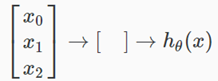Our input nodes (layer 1), also known as the "input layer", go into another node (layer 2), which finally outputs the hypothesis function, known as the "output layer".

We can have intermediate layers of nodes between the input and output layers called the "hidden layers."

In this example, we label these intermediate or "hidden" layer nodes a02..........an2 and call them "activation units."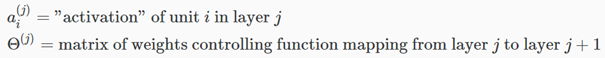If we had one hidden layer, it would look like: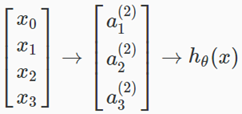The values for each of the "activation" nodes is obtained as follows: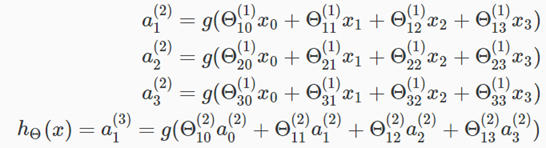This is saying that we compute our activation nodes by using a 3×4 matrix of parameters. We apply each row of the parameters to our inputs to obtain the value for one activation node. Our hypothesis output is the logistic function applied to the sum of the values of our activation nodes, which have been multiplied by yet another parameter matrix θ(2) containing the weights for our second layer of nodes

Each layer gets its own matrix of weights, θ(j).

The dimensions of these matrices of weights is determined as follows: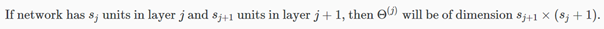The +1 comes from the addition in θ(j) of the "bias nodes," x0 and θ0(j). In other words the output nodes will not include the bias nodes while the inputs will. The following image summarizes our model representation: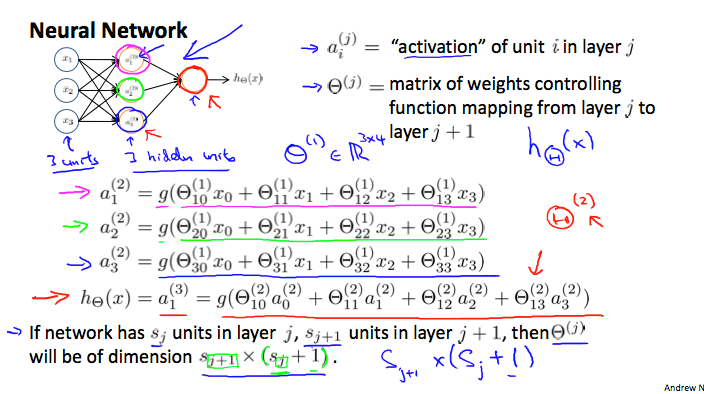Example: layer 1 has 2 input nodes and layer 2 has 4 activation nodes. Dimension of θ(1) is going to be 4×3 where sj=2 and sj+1=4, so × (sj+1)=4×3.

## b. Model representation 2:

To re-iterate, the following is an example of a neural network: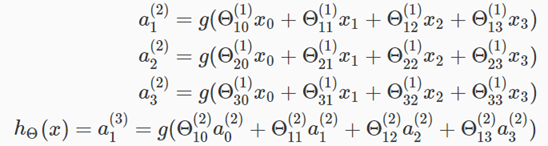In this section we'll do a vectorized implementation of the above functions. We're going to define a new variable zk(j) that encompasses the parameters inside our g function. In our previous example if we replaced by the variable z for all the parameters we would get: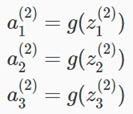In other words, for layer j=2 and node k, the variable z will be: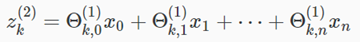The vector representation of x and zj is: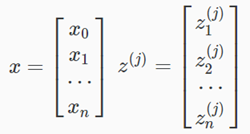Setting x = a(1) , we can rewrite the equation as: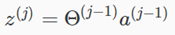We are multiplying our matrix θ(j-1) with dimensions sj × (n+1)  (where  is the number of our activation nodes) by our vector aj-1 with height (n+1). This gives us our vector zj with height sj. Now we can get a vector of our activation nodes for layer j as follows: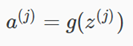Where our function g can be applied element-wise to our vector zj.

We can then add a bias unit (equal to 1) to layer j after we have computed a(j). This will be element a0(j) and will be equal to 1. To compute our final hypothesis, let's first compute another z vector: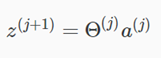We get this final z vector by multiplying the next theta matrix after θ(j-1) with the values of all the activation nodes we just got. This last theta matrix θ(j) will have only one row which is multiplied by one column a(j) so that our result is a single number. We then get our final result with: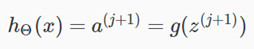Notice that in this last step, between layer j and layer j+1, we are doing exactly the same thing as we did in logistic regression. Adding all these intermediate layers in neural networks allows us to more elegantly produce interesting and more complex non-linear hypotheses.

## i. Intuition 1:

A simple example of applying neural networks is by predicting x1 AND x2, which is the logical 'and' operator and is only true if both x1 and x2 are 1.

The graph of our functions will look like: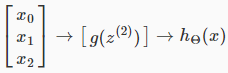Remember that xis our bias variable and is always 1.

Let's set our first theta matrix as: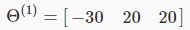This will cause the output of our hypothesis to only be positive if both x1 and x2 are 1. In other words: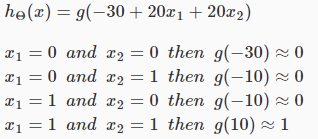So we have constructed one of the fundamental operations in computers by using a small neural network rather than using an actual AND gate. Neural networks can also be used to simulate all the other logical gates. The following is an example of the logical operator 'OR', meaning either x1 is true or x2  is true, or both: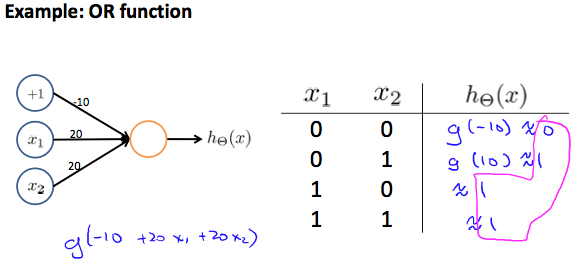Where g(z) is the following: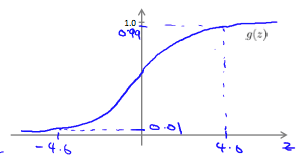## ii. Intuition 2:

The θ(1) matrices for AND, NOR, and OR are: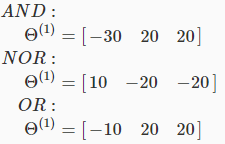We can combine these to get the XNOR logical operator (which gives 1 if x1 and x2 are both 0 or both 1).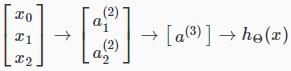For the transition between the first and second layer, we'll use a θ(1) matrix that combines the values for AND and NOR: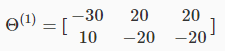For the transition between the second and third layer, we'll use a θ(2) matrix that uses the value for OR: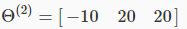Let's write out the values for all our nodes: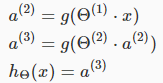And there we have the XNOR operator using a hidden layer with two nodes! The following summarizes the above algorithm: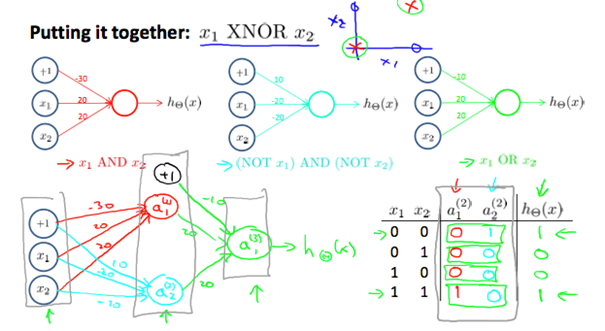## d. Multiclass Classification:

To classify data into multiple classes, we let our hypothesis function return a vector of values. Say we wanted to classify our data into one of four categories. We will use the following example to see how this classification is done. This algorithm takes as input an image and classifies it accordingly: